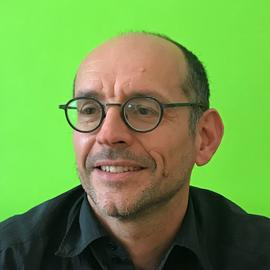# Talk "Full inference for the anisotropic fractional Brownian field" (joint work with Paul Escande).

### Abstract

The anisotropic fractional Brownian field is a non-stationary Gaussian field (Bonami and Estrade, 2003) which has been used for the modeling of image microtextures (Richard, 2016-18). Having stationary increments, its probability distribution is characterized by a semi-variogram whose spectral representation in polar coordinates

$$\forall x \in \mathbb{R}^d, v(x) = \frac{1}{2} \int_{-\pi/2}^{\pi/2} \tau(\theta) \left\vert \cos(\arg(x) - \theta) \right\vert^{2\beta(\theta)}\vert x \vert^{2\beta(\theta)} d\theta$$

is determined by two non-negative $\pi$-periodic functions $\tau$ and $\beta$ called the topothesy and the Hurst functions, respectively. In this talk, we focus on the issue of estimating these two functions from a single realization of the field.

Solving that issue is a key point for the characterization and the classification of image textures. It would also pave the way to the simulation of realistic textures from the field model. In the literature, this issue has been partially tackled in two different studies. In (Biermé and Richard, 2008), a method was built upon the Radon transform of the field to estimate the Hurst function. But, due to discretization issues, its application is restricted to a few directions $\theta$ and leads to inaccurate results. In (Richard, 2018), an inverse problem was stated and solved to estimate the topothesy in directions where the Hurst function is minimal.

In this talk, we will present a method for the estimation of the whole topothesy and Hurst functions. This method is based on a turning-band field which was initially proposed for the simulation of anisotropic fractional Brownian field (Biermé, Moisan and Richard, 2015), and whose semi-variogram approximates the one of an anisotropic fractional Brownian field. We will set an optimization problem to fit the semi-variogram of the turning-band field to the empirical semi-variogram. This problem is formulated as a non-linear separable least square problem (Golub, 2003). We then use a variable projection method to design an algorithm to solve numerically the problem. The design of this algorithm also includes a multigrid approach to improve the convergence. Eventually, we will present a numerical study of the performances of this algorithm on textures generated by the python package PyAFBF.

Date
Jun 7, 2022 5:06 PM — Jun 9, 2022 10:00 PM
Location
Laboratoire Paul Painlevé, Villeneuve d’Ascq##### Frédéric J.P. Richard
###### Professor of Applied Mathematics

Professor of applied mathematics whose research interests include image processing, spatial statistics and data science.